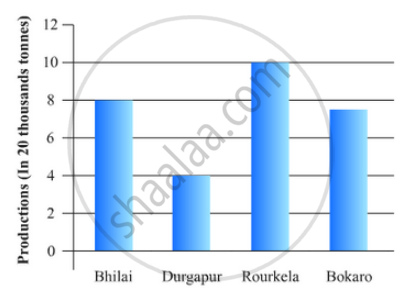# The Production of Saleable Steel in Some of the Steel Plants Our Country During 1999 is Given Below: - Mathematics

The production of saleable steel in some of the steel plants our country during 1999 is given below:

 Plant Bhilai Durgapur Rourkela Bokaro Production(In thousand tonnes) 160 80 200 150

Construct a bar graph to represent the above data on a graph paper by using the scale 1 big divisions = 20 thousand tonnes.

#### Solution

To represent the given data by a vertical bar graph, we first draw horizontal and vertical axes. Let us consider that the horizontal and vertical axes represent the plants and the production in thousand tonnes respectively. We have to draw 4 bars of different lengths given in the table.

The scale 1 big divisions must be 20 thousand tonnes. So, first find the heights of the bars corresponding to different plants. After that, we follow the well known procedure.

The heights of the different bars are:

1. The height of the bar corresponding to Bhilai is 160/20=8 big division.

2. The height of the bar corresponding to Durgapur is 80/20=4  big divisions.

3. The height of the bar corresponding to Rourkela is 200/20=10 big divisions.

4. The height of the bar corresponding to Bokaro is 150/20=7.5  big divisions.

At first we mark 4 points in the horizontal axis at equal distances and erect rectangles of the same width at these points. The heights of the rectangles are proportional to the productions.

The vertical bar graph of the given data is following:Note that the one big division in the vertical axis is equivalent to 20 thousand tonnes.

Concept: Graphical Representation of Data
Is there an error in this question or solution?
Chapter 23: Graphical Representation of Statistical Data - Exercise 23.2 [Page 25]

#### APPEARS IN

RD Sharma Mathematics for Class 9
Chapter 23 Graphical Representation of Statistical Data
Exercise 23.2 | Q 5 | Page 25

Share